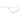# Maximum Subarray Problem

May 22, 2019# Problem Statement

You are given an array of integers. And, you have find the range of indexes or sub-array from original array whose sum is maximum in whole array.

Example:

``````2 -4 1 -3 2 3 -8

# Maximum sum = 5
# Indexes = [4,5]``````

Note: The input is a combination of negative and positive integers. If all numbers are positive, the answer will just be the sum of all the elements. And similarly, if all the numbers are negative. The answer will be the minimum negative number.

You can simply put two loops, and start calculating sum. Keep a track of max sum and indexes.

``````public static void calculate_bad(int[] a) {
int maxSum = Integer.MIN_VALUE;
int lIndex = 0;
int rIndex = 0;
int len = a.length;

for (int i=0; i<len; i++) {
int sum = 0;
for (int j=i; j<len; j++) {
sum += a[j];
if (sum > maxSum) {
maxSum = sum;
lIndex = i;
rIndex = j;
}
}
}

//maxSum, i, j is the result
}``````

In the above solution, note the 2nd loop where I’m starting with the index from outer loop, thus giving the oppurtunity of testing out every combination. The running time is O(n^2). Lets see an optimal solution.

More optimal solution can be of complexity: O(n log n), OR O(n)

## O(n log n) solution to Max sub-array problem

This algorithm is again based on Divide and Conquer approach. We find a mid element in array, which divides array in two equal halves.

Now, there can be three different sums:

1. From left sub-array
2. From right sub-array
3. sum of array crossing that mid point.

And, we are able to calculate above three sums. We just have to find the maximum of three.

Lets look at the code.

``````public static class MaxSubarrayData {
private int lindex;
private int rindex;
private int sum;

//and a bunch of getter-setter methods
}
public static MaxSubarrayData calculate_good_crossing(int[] arr, int l, int m, int r) {

MaxSubarrayData res = new MaxSubarrayData(l, r, 0);

int maxLeft = 0;
int sum = 0;
for (int i=m; i>=l; i--) {
sum += arr[i];
if (sum > maxLeft) {
maxLeft = sum;
res.setLindex(i);
}
}

int maxRight = 0;
sum = 0;
for (int i=m+1; i<=r; i++) {
sum += arr[i];
if (sum > maxRight) {
maxRight = sum;
res.setRindex(i);
}
}

res.setSum(maxLeft + maxRight);
return res;
}

public static MaxSubarrayData calculate_good(int[] arr, int l, int r) {
if (l == r) {
return new MaxSubarrayData(l, r, arr[l]);
}
else {
int m = (l+r)/2;
MaxSubarrayData left = calculate_good(arr, l, m);
MaxSubarrayData right = calculate_good(arr, m+1, r);

MaxSubarrayData crossing = calculate_good_crossing(arr, l, m, r);

if (left.getSum() > right.getSum() && left.getSum() > crossing.getSum()) {
return left;
}
else if (right.getSum() > left.getSum() && right.getSum() > crossing.getSum()) {
return right;
}
else {
return crossing;
}
}
}``````

In method calculate_good_crossing, the crux is that we need to find sum from mid to left index, and then mid to right index.

## Best Optimized algorithm

There is one algorithm which takes O(n). Yes, you heared it right. The algorithm is so simple and efficient that it runs in O(n) time complexity.

``````public static MaxSubarrayData calculate_best(int[] arr) {
MaxSubarrayData res = new MaxSubarrayData(0, 0, arr);

int maxSum = 0;
int sum = 0;
int len = arr.length;
for (int i=0; i<len; i++) {
sum += arr[i];

if (sum > maxSum) {
maxSum = sum;

res.setRindex(i);
res.setSum(maxSum);
}
if (sum < 0) {
sum = 0;
res.lindex = i+1;
}
}

return res;
}``````

The key here is that you keep a track of the maximum. And, one more variable who just keeps on adding the numbers, and reset to zero if the sum goes below 0. i.e. what is the need of having a sum less than zero.